# Defensive Forecasting

Defensive forecasting is a technique for competitive on-line prediction based on game-theoretic probability.

## Basic principle

A typical law of game-theoretic probability (see, e.g., the strong law of large numbers for bounded observations) says that either some interesting property of the forecasts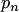and outcomes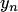(such as their unbiasedness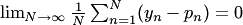) holds or Sceptic's capital tends to infinity. Typically, the strategy for Sceptic implicit in such a law is continuous. The basic observation (made by Takemura in 2004; first published in Vovk et al. 2005) is that, if Sceptic is known to follow a continuous strategy, Forecaster can play in such a way as to prevent Sceptic's capital from growing. For example, adding signals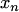to the protocol used in the strong law of large numbers for binary observations and making Sceptic announce his strategy for each round at the beginning of that round, we obtain the following protocol:

Players: Skeptic, Forecaster, Reality
Protocol:Initialize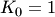FOR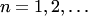:Reality announces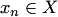Sceptic announces continuous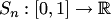Forecaster announces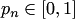Reality announces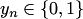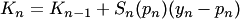END.

The usual restriction on Sceptic that his capital should always be nonnegative (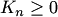for all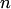) becomes irrelevant in defensive forecasting.

Basic Lemma. Forecaster has a strategy in the above protocol that ensures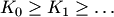.

The proof is trivial: Forecaster's is chosen as a root of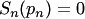if one exists, or as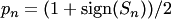if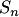has no roots in the interval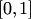.

The Basic Lemma can be generalized to a wide class of forecasting games. Therefore, for a typical continuous law of game-theoretic probability Forecaster has a strategy that guarantees that the corresponding property will hold for the realized sequence of forecasts and outcomes. The corresponding strategy for Forecaster is said to have been obtained by the method of defensive forecasting.

In particular, one can apply defensive forecasting to the property of calibration-cum-resolution, which leads to the K29 algorithm. In some applications, such as those to competitive on-line prediction described below, the property of calibration-cum-resolution is replaced by the conjunction of calibration and resolution.

## Application to competitive on-line prediction

We would like to compete with prediction strategies indexed by. Let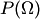be the set of all probability measures on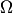and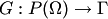be a choice function: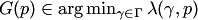,

where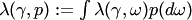,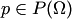. If the probability forecasts are chosen by defensive forecasting for suitable strategies for Sceptic, we will have: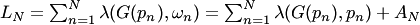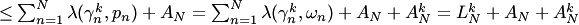,

where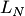is Learner's loss over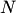steps,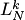is theth strategy's loss oversteps,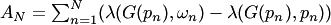is small, and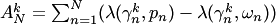is also small. The smallness of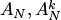(as compared with) will be ensured if defensive forecasting is applied to suitable forms of the law of large numbers.

The chain of equalities and inequalities given above says, in effect, that real losses are close to the (one-step-ahead conditional) expected losses. This part is a defensive forecasting part of the proof. The expected loss of Learner cannot exceed the expected loss of any of the strategies by the definition of a choice function. The choice funtion also tranforms the probability forecasts of defensive forecasting into real forecasts. Instead of existence of the actual choice function Vovk (2007) proves the existence of the approximate choice function.

In each particular case one should estimate the numbers. The inherent accuracy given by the law of large numbers is at best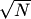, and so this technique cannot provide a regret term better than, the difference between the expected and real losses. There is Shortcut Defensive Forecasting for finitely many experts that allows one to replacewith smaller terms.

The technique of defensive forecasting can also be applied to infinite (and uncountable) classes of prediction rules, in particular reproducing kernel Hilbert spaces and proper functional Banach spaces.

## Two approaches to defensive forecasting

The well-known example due to Oakes (simplified by Dawid) imposes severe limitations on the possibility of well-calibrated forecasting. There are two known ways to overcome difficulties pointed out by Oakes and Dawid:

• assuming that the strategy for Sceptic is continuous;
• using randomization.

This article concentrated on the first approach. Both approaches have also been widely studied in von Mises's version of game-theoretic probability and in measure-theoretic probability (Foster, Vohra, Young, Sandroni, and many other people).

### Bibliography

• Vladimir Vovk, Akimichi Takemura, and Glenn Shafer. Defensive forecasting. In: Proceedings of the 10th International Workshop on Artificial Intelligence and Statistics (ed. by R. G. Cowell and Z. Ghahramani), pp. 365 – 372. Society for Artificial Intelligence and Statistics, 2005.
• Vladimir Vovk. On-line regression competitive with reproducing kernel Hilbert spaces (extended abstract). In: Theory and Applications of Models of Computation. Proceedings of the Third Annual Conference on Computation and Logic (ed. by J.-Y. Cai, S. B. Cooper and A. Li), Lecture Notes in Computer Science, vol. 3959, pp. 452 – 463. Berlin: Springer, 2006.
• Vladimir Vovk. Predictions as statements and decisions. arXiv:cs/0606093v1 [cs.LG], 2007